# Python | Decision Tree Regression using sklearn

Decision Tree is a decision-making tool that uses a flowchart-like tree structure or is a model of decisions and all of their possible results, including outcomes, input costs and utility.

Decision-tree algorithm falls under the category of supervised learning algorithms. It works for both continuous as well as categorical output variables.

The branches/edges represent the result of the node and the nodes have either:

1. Conditions [Decision Nodes]
2. Result [End Nodes]

The branches/edges represent the truth/falsity of the statement and takes makes a decision based on that in the example below which shows a decision tree that evaluates the smallest of three numbers: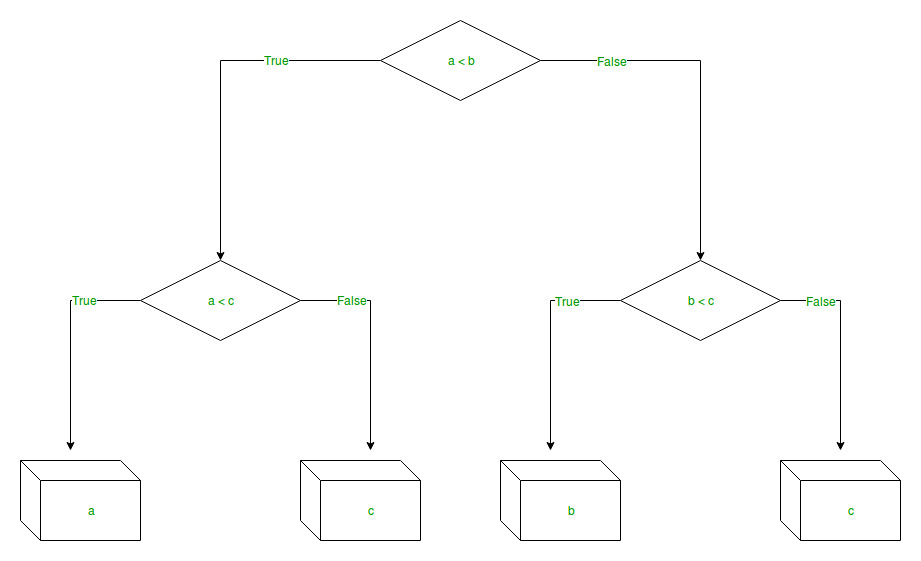Decision Tree Regression:
Decision tree regression observes features of an object and trains a model in the structure of a tree to predict data in the future to produce meaningful continuous output. Continuous output means that the output/result is not discrete, i.e., it is not represented just by a discrete, known set of numbers or values.

Discrete output example: A weather prediction model that predicts whether or not there’ll be rain in a particular day.
Continuous output example: A profit prediction model that states the probable profit that can be generated from the sale of a product.

Here, continuous values are predicted with the help of a decision tree regression model.

Let’s see the Step-by-Step implementation –

• Step 1: Import the required libraries.

 `# import numpy package for arrays and stuff ` `import` `numpy as np  ` ` `  `# import matplotlib.pyplot for plotting our result ` `import` `matplotlib.pyplot as plt ` ` `  `# import pandas for importing csv files  ` `import` `pandas as pd  `

• Step 2: Initialize and print the Dataset.

 `# import dataset ` `# dataset = pd.read_csv('Data.csv')  ` `# alternatively open up .csv file to read data ` ` `  `dataset ``=` `np.array( ` `[[``'Asset Flip'``, ``100``, ``1000``], ` `[``'Text Based'``, ``500``, ``3000``], ` `[``'Visual Novel'``, ``1500``, ``5000``], ` `[``'2D Pixel Art'``, ``3500``, ``8000``], ` `[``'2D Vector Art'``, ``5000``, ``6500``], ` `[``'Strategy'``, ``6000``, ``7000``], ` `[``'First Person Shooter'``, ``8000``, ``15000``], ` `[``'Simulator'``, ``9500``, ``20000``], ` `[``'Racing'``, ``12000``, ``21000``], ` `[``'RPG'``, ``14000``, ``25000``], ` `[``'Sandbox'``, ``15500``, ``27000``], ` `[``'Open-World'``, ``16500``, ``30000``], ` `[``'MMOFPS'``, ``25000``, ``52000``], ` `[``'MMORPG'``, ``30000``, ``80000``] ` `]) ` ` `  `# print the dataset ` `print``(dataset)  `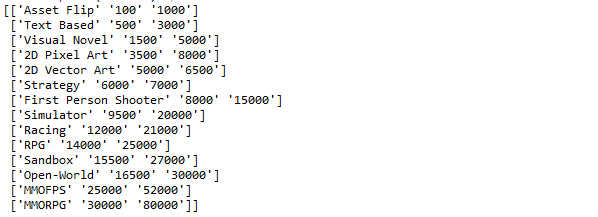• Step 3: Select all the rows and column 1 from dataset to “X”.

 `# select all rows by : and column 1 ` `# by 1:2 representing features ` `X ``=` `dataset[:, ``1``:``2``].astype(``int``)  ` ` `  `# print X ` `print``(X) `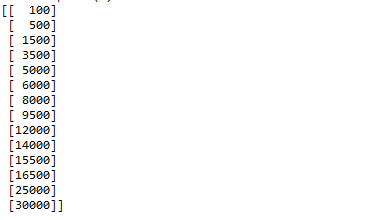• Step 4: Select all of the rows and column 2 from dataset to “y”.

 `# select all rows by : and column 2 ` `# by 2 to Y representing labels ` `y ``=` `dataset[:, ``2``].astype(``int``)  ` ` `  `# print y ` `print``(y) `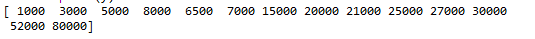• Step 5: Fit decision tree regressor to the dataset

 `# import the regressor ` `from` `sklearn.tree ``import` `DecisionTreeRegressor  ` ` `  `# create a regressor object ` `regressor ``=` `DecisionTreeRegressor(random_state ``=` `0``)  ` ` `  `# fit the regressor with X and Y data ` `regressor.fit(X, y) `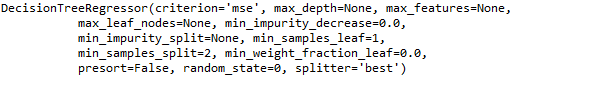• Step 6: Predicting a new value

 `# predicting a new value ` ` `  `# test the output by changing values, like 3750 ` `y_pred ``=` `regressor.predict(``3750``) ` ` `  `# print the predicted price ` `print``(``"Predicted price: % d\n"``%` `y_pred)  `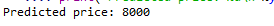• Step 7: Visualising the result

 `# arange for creating a range of values  ` `# from min value of X to max value of X  ` `# with a difference of 0.01 between two ` `# consecutive values ` `X_grid ``=` `np.arange(``min``(X), ``max``(X), ``0.01``) ` ` `  `# reshape for reshaping the data into  ` `# a len(X_grid)*1 array, i.e. to make ` `# a column out of the X_grid values ` `X_grid ``=` `X_grid.reshape((``len``(X_grid), ``1``))  ` ` `  `# scatter plot for original data ` `plt.scatter(X, y, color ``=` `'red'``) ` ` `  `# plot predicted data ` `plt.plot(X_grid, regressor.predict(X_grid), color ``=` `'blue'``)  ` ` `  `# specify title ` `plt.title(``'Profit to Production Cost (Decision Tree Regression)'``)  ` ` `  `# specify X axis label ` `plt.xlabel(``'Production Cost'``) ` ` `  `# specify Y axis label ` `plt.ylabel(``'Profit'``) ` ` `  `# show the plot ` `plt.show() `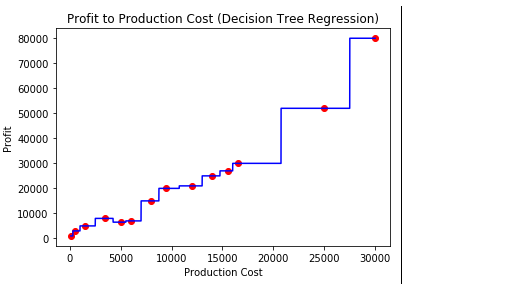• Step 8: The tree is finally exported and shown in the TREE STRUCTURE below, visualized using http://www.webgraphviz.com/ by copying the data from the ‘tree.dot’ file.

 `# import export_graphviz ` `from` `sklearn.tree ``import` `export_graphviz  ` ` `  `# export the decision tree to a tree.dot file ` `# for visualizing the plot easily anywhere ` `export_graphviz(regressor, out_file ``=``'tree.dot'``, ` `               ``feature_names ``=``[``'Production Cost'``])  `

Output (Decision Tree):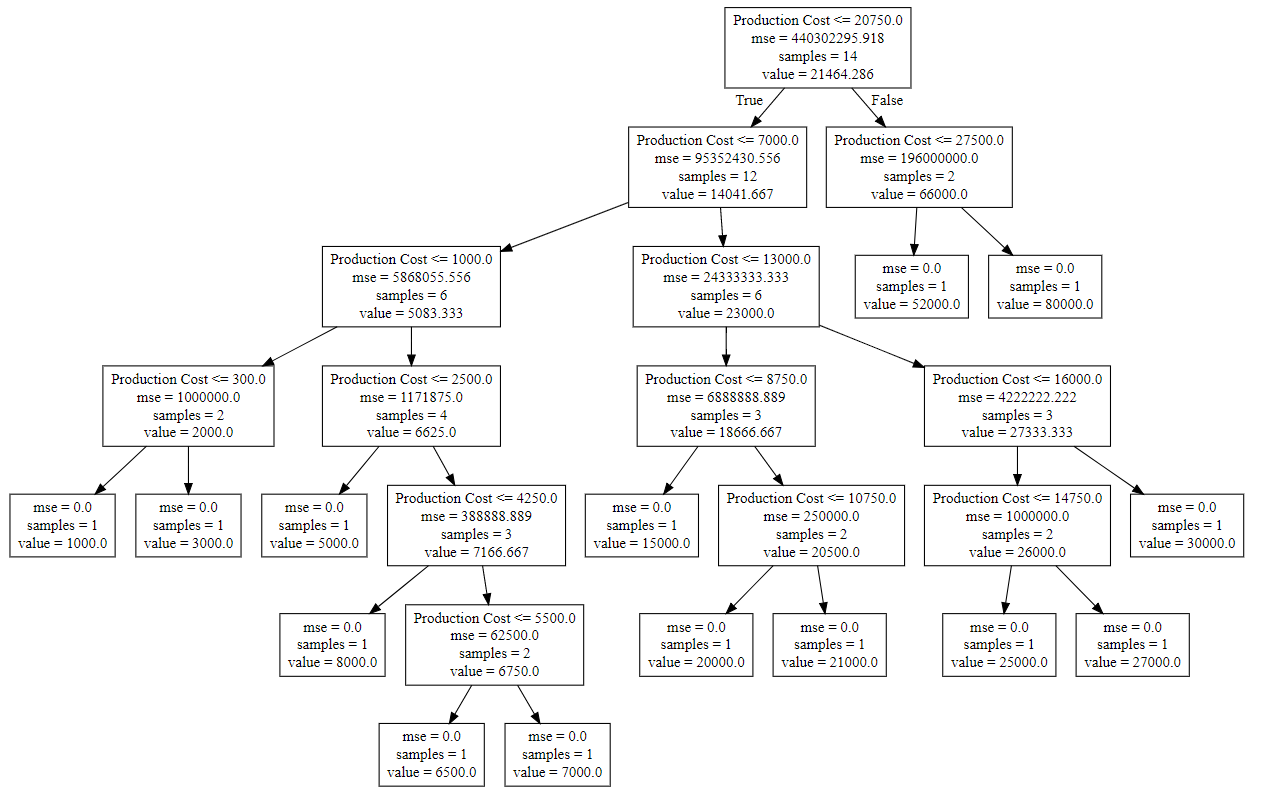Attention geek! Strengthen your foundations with the Python Programming Foundation Course and learn the basics.

To begin with, your interview preparations Enhance your Data Structures concepts with the Python DS Course.

My Personal Notes arrow_drop_upCheck out this Author's contributed articles.

If you like GeeksforGeeks and would like to contribute, you can also write an article using contribute.geeksforgeeks.org or mail your article to contribute@geeksforgeeks.org. See your article appearing on the GeeksforGeeks main page and help other Geeks.

Please Improve this article if you find anything incorrect by clicking on the "Improve Article" button below.